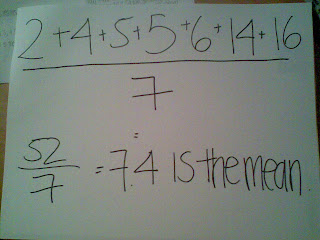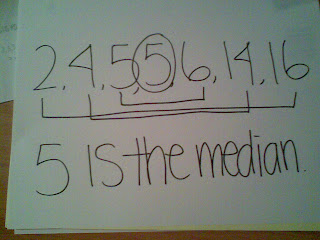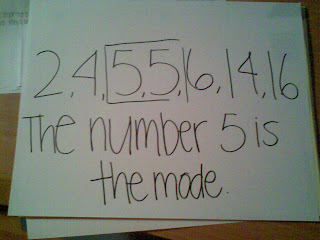### Michelle's Measures of Central Tendency

Monday, September 29, 2008Mean
• Arrange the data in ascending numerical order.
• The mean is the sum of all data

ian• Arrange the data in acending numerical order.
• The median is the number that is in the middle
• If there is two numbers in the middle number between those two numbers.Mode
• Arrange the data in ascending numerical order.
• The mode is the number that shows up often.Range
• Arrange the data in ascending order.
• The range is when you subtract the smallest piece of data from the largest piece of data.

Heres the video that explains mean, median, mode, and range.